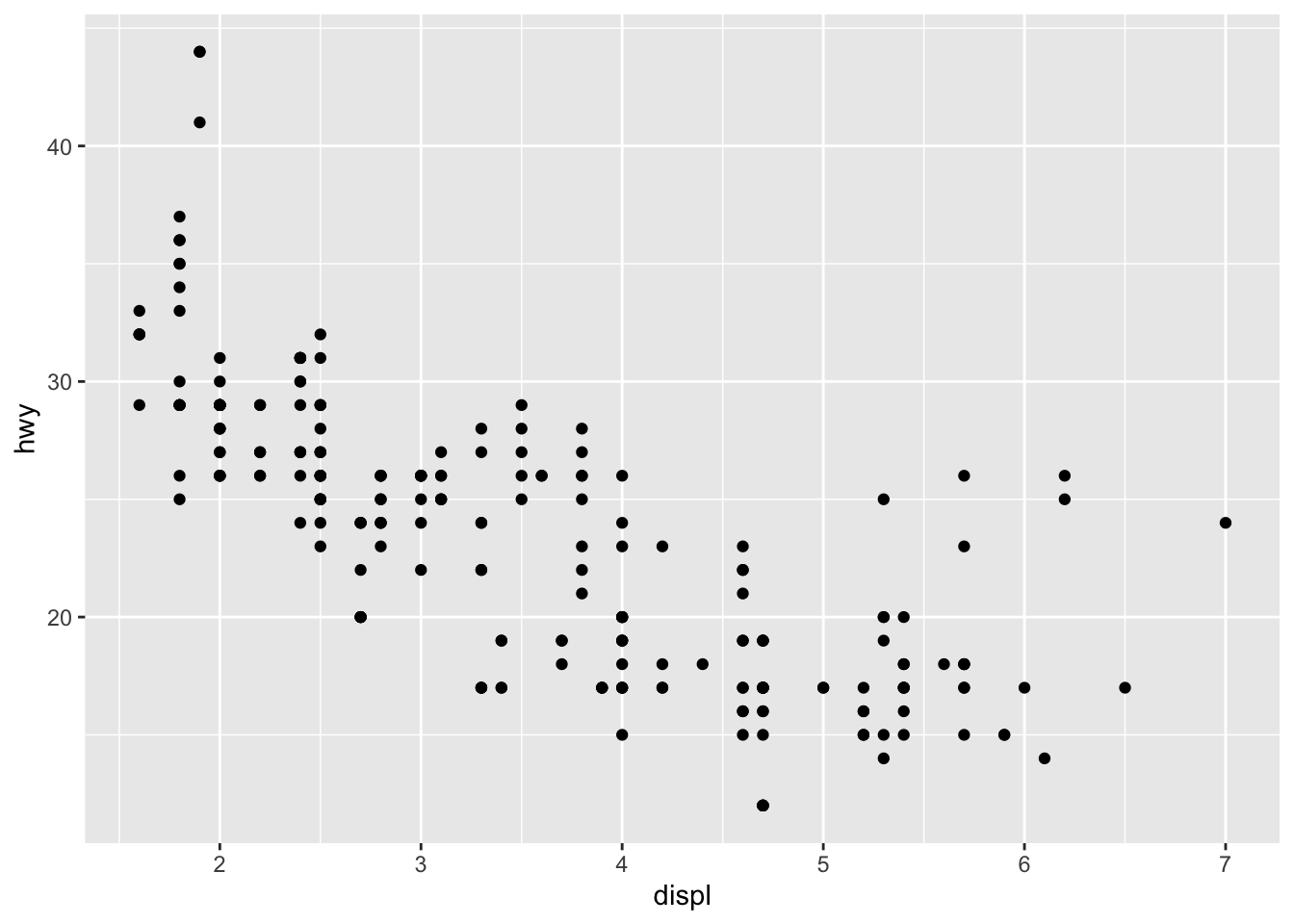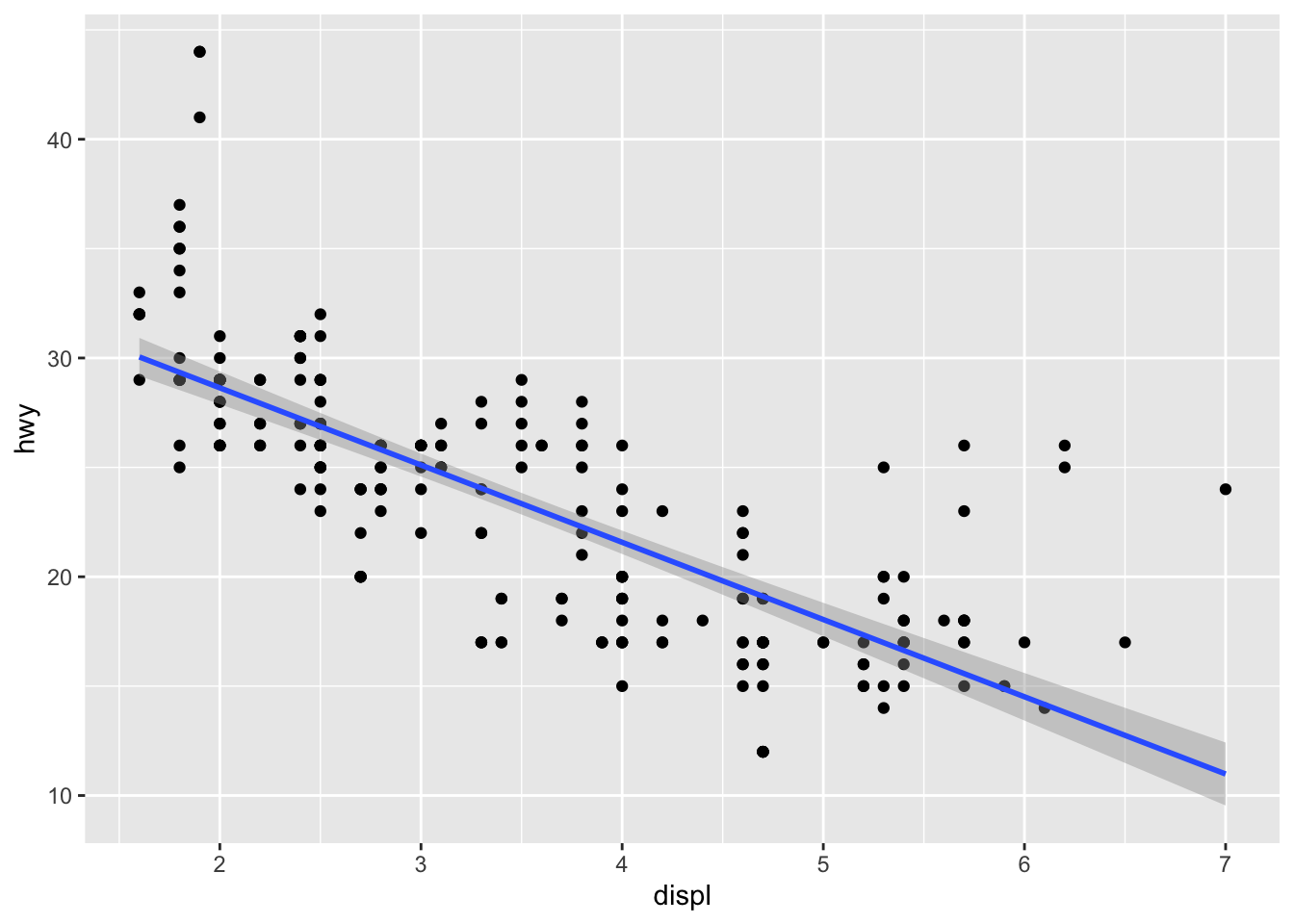## Visualizing Data

### Getting started

One particularly powerful aspect of R is that it enables one visualize data in a variety of ways. Additionally, it gives users a wide variety of customization options. We will work below on a number of common data visualizations. But rememeber, once you get the hang of the basics, the sky is the limit! Note that this tutorial is a simplified version of [this one] (https://r4ds.had.co.nz/data-visualisation.html). For more details on what is possible, check out the source webpages!

To get started, lets load ggplot and look at one of the datasets that comes with ggplot:

``````library(ggplot2)
summary(mpg)``````
``````##  manufacturer          model               displ            year
##  Length:234         Length:234         Min.   :1.600   Min.   :1999
##  Class :character   Class :character   1st Qu.:2.400   1st Qu.:1999
##  Mode  :character   Mode  :character   Median :3.300   Median :2004
##                                        Mean   :3.472   Mean   :2004
##                                        3rd Qu.:4.600   3rd Qu.:2008
##                                        Max.   :7.000   Max.   :2008
##       cyl           trans               drv                 cty
##  Min.   :4.000   Length:234         Length:234         Min.   : 9.00
##  1st Qu.:4.000   Class :character   Class :character   1st Qu.:14.00
##  Median :6.000   Mode  :character   Mode  :character   Median :17.00
##  Mean   :5.889                                         Mean   :16.86
##  3rd Qu.:8.000                                         3rd Qu.:19.00
##  Max.   :8.000                                         Max.   :35.00
##       hwy             fl               class
##  Min.   :12.00   Length:234         Length:234
##  1st Qu.:18.00   Class :character   Class :character
##  Median :24.00   Mode  :character   Mode  :character
##  Mean   :23.44
##  3rd Qu.:27.00
##  Max.   :44.00``````

As we can see from the summary, this dataset includes a number of characteristics of cars that might affect fuel efficiency (in miles per gallon for city or highway driving) from 1999 to 2008. Remember that we can get more detailed information about the dataset by using the “help” function in R:

``help(mpg)``

### Making our first plot: Scatterplots

First, I am going to make a simple prediction regarding the relationship between highway fuel efficiency (hwy) and engine size (displ). My hypothesis is that larger engines will have lower fuel efficiency. Lets see if this hypothesis appears to fit the data.

``````ggplot(data = mpg) + #this tells ggplot which dataset to use
geom_point(mapping = aes(x = displ, y = hwy)) #this sets the x and y axis and plots each data point``````As the plot indicates, there seems to be a negative relationship between highway fuel efficiency and engine size (as we hypothesized).

We can also add a line of best fit, which is used in the calculation of correlations. Note that adding layers to plots using ggplot is very simple. We only need to use the “+” symbol.

``````ggplot(data = mpg) + #this tells ggplot which dataset to use
geom_point(mapping = aes(x = displ, y = hwy)) + #this sets the x and y axis and plots each data point
geom_smooth(mapping = aes(x = displ, y = hwy), method = lm) #this creates the line of best fit``````To explore this dataset further, we can add more information to the plot. Next, we will make the points on the plot different colors based on the type of car.

``````ggplot(data = mpg) + #this tells ggplot which dataset to use
geom_point(mapping = aes(x = displ, y = hwy, color = class)) + #this sets the x and y axis and plots each data point, "color" is used here to differ the color of the dots based on the class of car (suv, etc.)
geom_smooth(mapping = aes(x = displ, y = hwy), method = lm, color = "black") #here, we set the color of the line explicitly to black``````